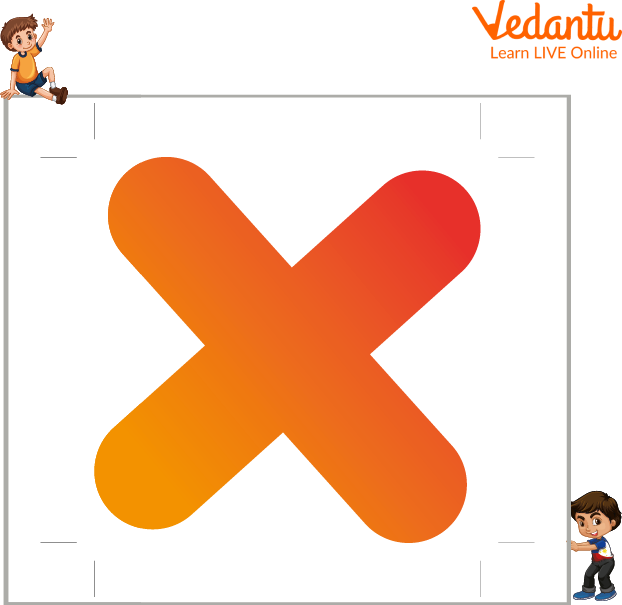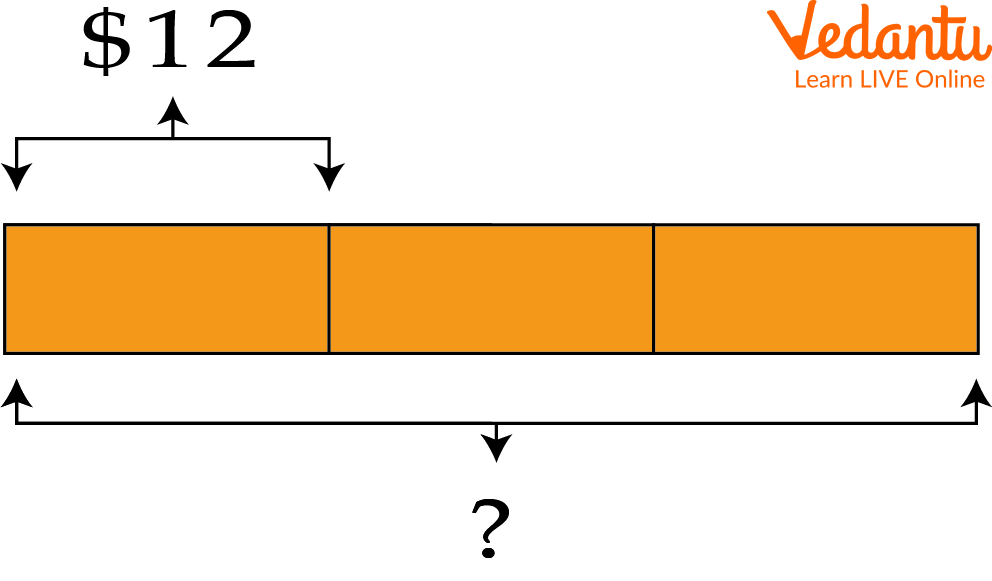Courses
Courses for Kids
Free study material
Offline Centres
More

# Multiplication StatementLast updated date: 01st Dec 2023
Total views: 98.1k
Views today: 1.98k## A Brief Explanation of Multiplication Statement

A multiplication statement is a mathematical statement that can be used to calculate the product of several given numbers. The statement of the multiplication theorem can be written in many different ways. Students need to know how to write each type for multiplication problem-solving.

Multiplication is an operation that represents the basic idea of repeated addition of the same number. The multiplied numbers are called the factors, and the result obtained after the multiplication of two or more numbers is known as the product of those numbers. Multiplication is used to simplify the task of repeated addition of the same number. In this article, we will look at a multiplication statement and how to write a multiplication statement.Multiplication Statement

## What is a Multiplication Statement?

The statement of a multiplication theorem is a mathematical equation that states how many objects are multiplied together to get a result. A multiplication statement in mathematics is an equation that states how many objects are multiplied together to get a result. For example, the equation $3 \times 2$ can be read as "three times two."

## How to Write a Multiplication Statement?

The multiplication statement is a sentence that explains how to write a multiplication statement. It is usually written as “multiply the first number by the second number.” When writing a multiplication statement, it is important to ensure you are clear about what you mean. The first way is to write the multiplication statement as an equation. The second way is to write the multiplication statement as a sentence. In mathematics, a multiplication statement is a statement that can be written in the form "$a \times b = c$"

It can be stated as the multiplication of a and b gives the product c.

Where a,b and c are variables.

## Solved Multiplication Statement Sums

Here is some solved multiplication statement sums:

Example: A table costs 12 dollars. How much do three of these tables cost?

Ans: Given a table of cost prices 12 dollars

So, for three such tables, the cost will become

$12 \times 3 = 36$

Thus, the cost of these three tables is 36 dollars.Multiplication Statement Problems

Example: In a single day, Amit delivers 405 books. In five days, how many books does he deliver?

Ans: As per the question, in a single day, Amit delivers 405 books

So to deliver such books, several days will be:

$405 \times 5 = 2025$

Thus, Amit delivered 2025 books in just 5 days.

## Multiplication Statement Problems for Practice

To build multiplication problem-solving skills, some multiplication statement problems are given as follows:

Q 1. A pack of pens contains 8 pens. How many pens are there in 6 packs?

Ans. 48 pens.

Q 2. A box of apples contains 150 apples. How many apples are there in 3 such boxes?

Ans. 450 apples.

Q 3. A dozen bananas consist of 12 bananas, then how many are there in 5 dozen?

Ans. 60 bananas.

## Summary

The statement multiplication theorem is a mathematical formula used to find the product of two or more numbers. In this article, we discussed the process of solving multiplication statement sums. We also looked at different approaches that can be taken to solve multiplication statement problems. The best way to deal with a multiplication statement is by using an approach involving grouping like terms together and multiplying them all together. We hope you have enjoyed this article and gained some understanding of multiplication statements and problem-solving. If you still have any questions, please feel free to ask.

## FAQs on Multiplication Statement

1. What are the benefits of using multiplication statements for mathematics?

The use of multiplication statements in mathematics is relatively recent. The use of these statements is beneficial to students and teachers. The benefits of using multiplication statements are that they are easy to understand and remember, they help students better visualise the concepts, and they make it easier for students to answer questions about math problems. In addition, these statements can also help with the understanding of algebraic equations.

2. How do the different types of multiplication statements work?

There are three types of multiplication statements:

1) The multiplicative identity,

2) The commutative law, and

3) The associative law.

3. How do you know when to use a multiplication statement?

A multiplication statement is a sentence that uses the word "times" to make a mathematical comparison. You should use a multiplication statement when you want to compare two things or when you want to show how much of one thing is equal to another. For example, The number of students in the class is times the number of desks in the classroom.